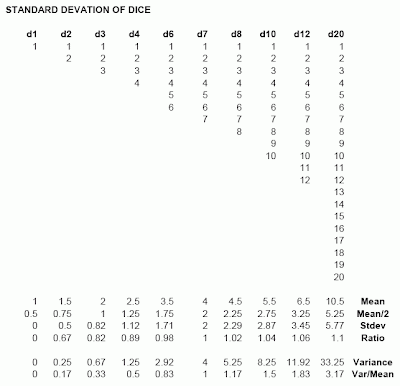## 2010-11-22

### Dice Statistics

Observation -- The standard deviation of a normal polyhedral die (i.e., a discrete uniform distribution 1..N with small N) is approximately one-half of the mean. For the case of a 7-sided die, the standard deviation is exactly equal to one-half of the mean. For dice with fewer sides it is less, and for more sides it is greater.

The d7 coincidentally also has the property that the variance exactly equals the mean. However, the two are not generally close for other sorts of dice (although still less for smaller dice, and more for bigger dice).1.I think there's something profound in there. But it beats me. :)

2.Hmm. It seems the d7 having the same mean and variance (4) is a coincidence of having the discrete uniform distribution going from 1 to n, rather than from, 1+x to n+x, where e.g., x=5 (uniform span of 6-12), and then the mean is 9 and the variance is 4. If the d7, d8, d23, d47, etc. were labeled by different conventions the convergence of mean and variance would occur elsewhere, right?

3.Yep. But they're not.